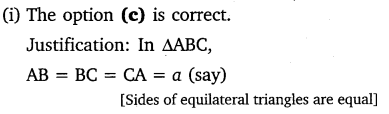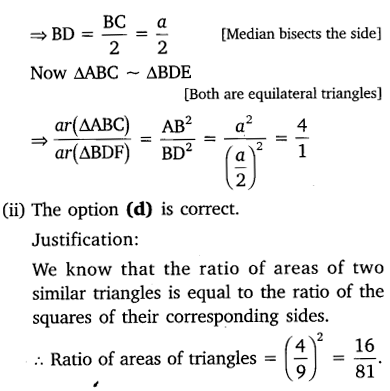# CLASS 10 MATH NCERT SOLUTIONS FOR CHAPTER – 6 TRIANGLES EX – 6.4

## Triangles

Ex 6.4 Class 10 Maths Question 1.
Let ∆ABC ~ ∆DEF and their areas be, respectively, 64 cm2 and 121 cm2. If EF = 15.4 cm, find BC.
Solution:
We have ∆ABC ~ ∆DEFQuestion 2.
Diagonals of a trapezium ABCD with AB || DC intersect each other at the point O. If AB = 2 CD, find the ratio of the areas of triangles AOB and COD.

Solution:
In the figure below, a trapezium ABCD is shown, in which AB || DC  and AB = 2DC. Its diagonals interest each other at the point O.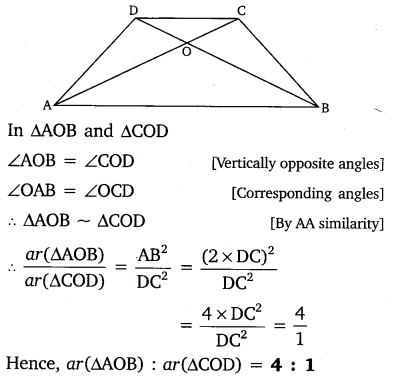Question 3.
In the given figure, ABC and DBC are two triangles on the same base BC. If AD intersects BC at O, show that:

Solution: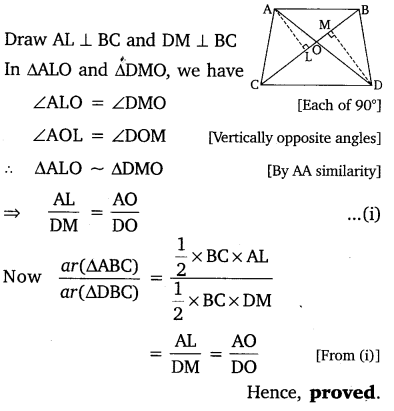Question 4.
If the areas of two similar triangles are equal, prove that they are congruent.

Solution: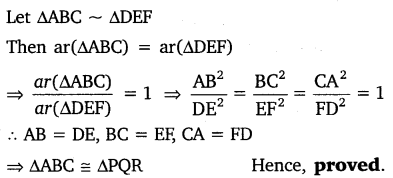Question 5.
D, E and F are respectively the mid-points of sides AB, BC and CA of ∆ABC. Find the ratio of the areas of ∆DEF and ∆ABC.

Solution: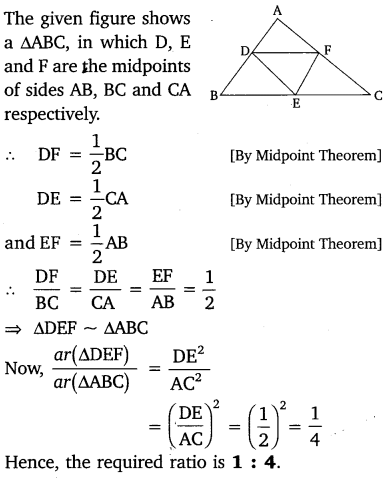Question 6.
Prove that the ratio of the areas of two similar triangles is equal to the square of the ratio of their corresponding medians.

Solution: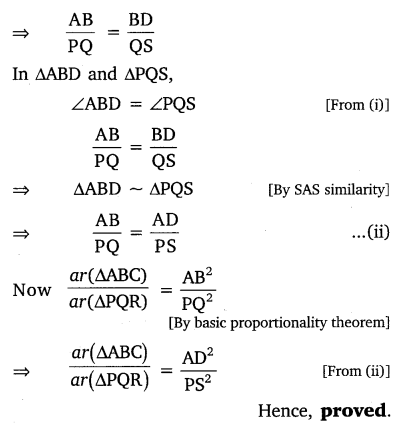Question 7.
Prove that the area of an equilateral triangle described on one side of a square is equal to half the area of the equilateral triangle described on one of its diagonals.

Solution: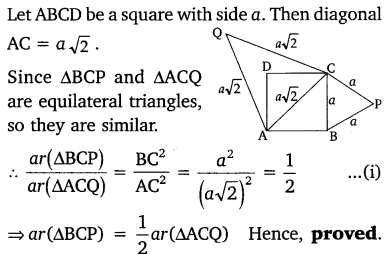Question 8.
Tick the correct answer and justify

(i) ABC and BDE are two equilateral triangles such that D is the mid-point of BC. Ratio of the areas of triangles ABC and BDE is
(a) 2:1
(b) 1:2
(c) 4:1
(d) 1:4

(ii) Sides of two similar triangles are in the ratio 4:9. Areas of these triangles are in the ratio
(a) 2 :3
(b) 4:9
(c) 81:16
(d) 16:81

Solution: